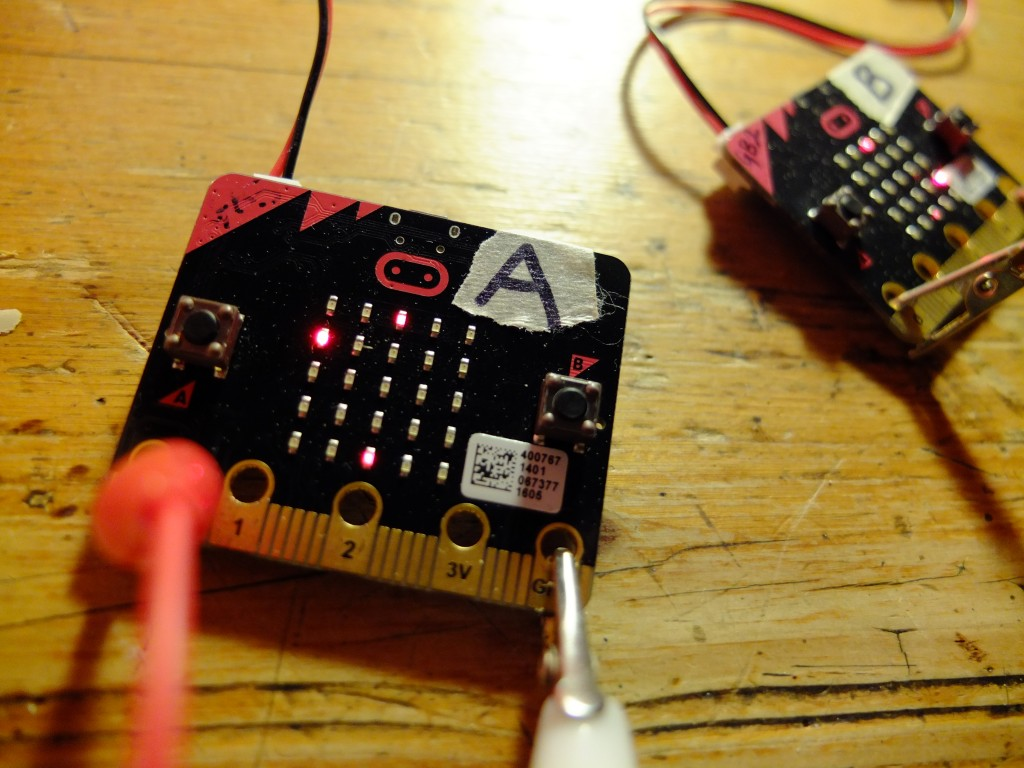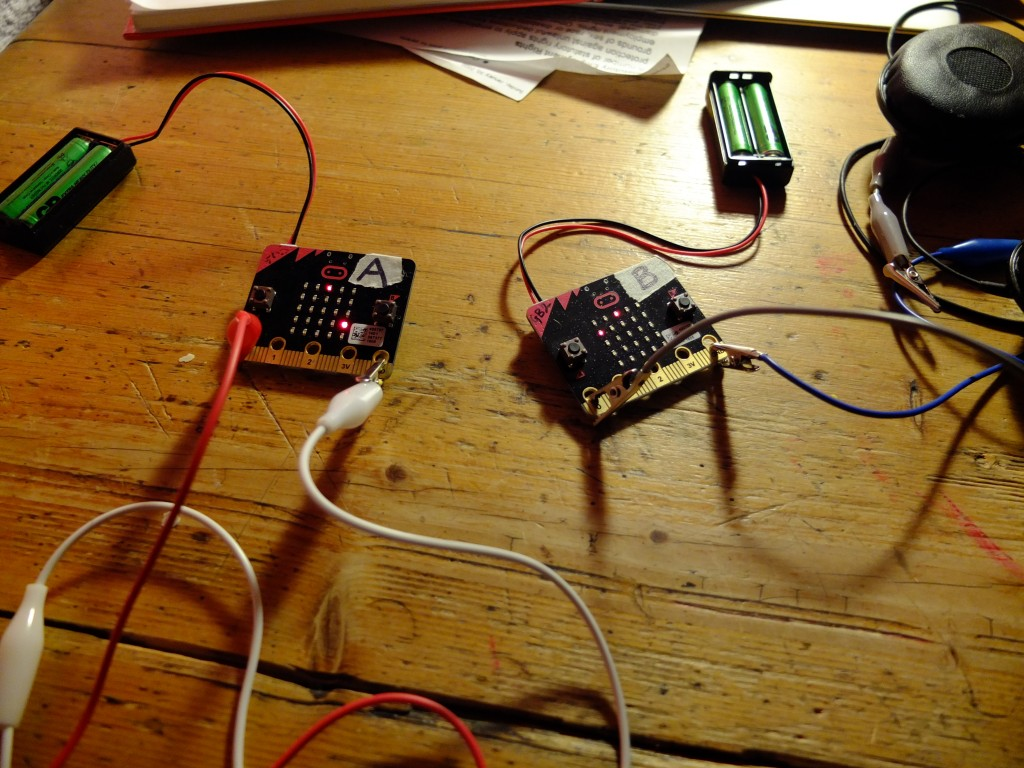# Pongo: wireless microbit Python pongI love the wireless capabilities of Python on the BBC microbit and I’ve been using it with some success in my Year 8 classes.

I thought I’d have a go at writing a wireless Pong game in Python – it took me a lot longer than I expected for various reasons. I really wanted to have the same code running on both microbits, but I soon abandoned that as too complex. Much easier to have one microbit – Player A – controlling the game and deciding who gets a point and when. Player B is the ‘slave’ only sending its left and right paddle moves back to Player A and mirroring (literally) Player A’s screen.

I was keen to have each screen the same – rather than extending a long screen like a wired version I’ve seen. This is because I want each player to be able to be quite far apart, so seeing the other player’s screen isn’t necessary.

How to play

Flash Player A code (below) on to one Microbit using Mu, and Player B on to a separate microbit. You can optionally connect a headphone or buzzer to pins 0 and 1 on each microbit for some audio feedback joy.

Power up Player B first – it will wait for messages from Player A. Then power up Player A. The game starts straight away with the ball – the bright dot in the middle of the screen – moving in a random direction. Move your paddle left and right using A and B buttons. If you fail to hit the ball when it reaches your end the other player gets a point (points tallies are not shown on the screen) and the first player to 5 points wins. To play again you both need to press the reset button on the back of the microbits.

How it works

Player B is the easy one to explain. It runs a loop constantly polling for messages and keypresses. If you press button A to move left, or B to move right, it sends a message with your bat’s new position. It also listens for different kinds of messages from player A’s microbit. They all start with different code letters:
p + a number is the position of player A’s bat.
x and y messages give the current location of the ball, which is then inverted using a dictionary look-up table called ‘`bat_map`‘.
a and b messages give the respective scores or player A and B.

If a player reaches the winning score (5) it breaks out of the loop and plays a happy song (Nyan cat) if player B has won and a sad song (funeral march) is player A has won.

Player A is the master controller. It picks a random direction for the ball to start moving and bounces the ball if it hits any of the sides. If it hits the top or bottom and a player’s bat isn’t in the way, the other player gets a point. It has a crude timer using variables `counter` and `delay` – every time it reaches 1000 it moves the ball (I couldn’t work out how to get proper timers to work in Microbit Python – if indeed this is possible). If a player hits the ball with their bat it speeds up a bit.

It sends messages (as described above) to Player B with the ball position, score and player A bat position. The game ends in the same way as player B’s code described above, except you get the happy tune if player A wins and the sad one if player B wins.

How to mod

You can make the game faster by making the value of `delay` smaller. You can also make it last longer by increasing the value of `winning_score` in both sets of code.

A nice extension would be to add more sound (when you hit the ball for example) and to add levels with the game getting faster each time someone wins a game.

Let me know how you get on with it and if you have any other ideas for improvements – the physics of the ball bouncing is one area that I could do with help on!Here’s the player A code:

```# Pongo by @blogmywiki
# player A code - with points

import random
from microbit import *
from music import play, POWER_UP, JUMP_DOWN, NYAN, FUNERAL

a_bat = 2              # starting position of player A bat
b_bat = 2              # starting position of player B bat
bat_map = {0: 4, 1: 3, 2: 2, 3: 1, 4: 0}
ball_x = 2             # starting position of ball
ball_y = 2
directions = [1, -1]   # pick a random direction for ball at start
x_direction = random.choice(directions)
y_direction = random.choice(directions)
delay = 1000           # used as a crude timer
counter = 0            # used as a crude timer
a_points = 0
b_points = 0
winning_score = 5
game_over = False

def move_ball():
global ball_x, ball_y, x_direction, y_direction, counter, a_bat, b_bat, a_points, b_points, delay
display.set_pixel(ball_x, ball_y, 0)
ball_x = ball_x + x_direction
ball_y = ball_y + y_direction
if ball_x < 0:							# bounce if hit left wall
ball_x = 0
x_direction = 1
if ball_x > 4:							# bounce if hit right wall
ball_x = 4
x_direction = -1
if ball_y == 0:
if ball_x == b_bat:					# bounce if player B hit ball
ball_y = 0
y_direction = 1
delay -= 50						# speed up after bat hits
else:
play(POWER_UP, wait=False)      # A gets point if B missed ball
a_points += 1
ball_y = 0
y_direction = 1

if ball_y == 4:							# bounce if player A hits ball
if ball_x == a_bat:
ball_y = 4
y_direction = -1
delay -= 50						# speed up after bat hits
else:
play(JUMP_DOWN, wait=False)     # player B gets point if A misses
b_points += 1
ball_y = 4
y_direction = -1
counter = 0

while not game_over:
counter += 1
display.set_pixel(a_bat, 4, 6)        # draw bats
display.set_pixel(b_bat, 0, 6)
display.set_pixel(ball_x, ball_y, 9)  # draw ball
if button_a.was_pressed():
display.set_pixel(a_bat, 4, 0)
a_bat = a_bat - 1
if a_bat < 0:
a_bat = 0
if button_b.was_pressed():
display.set_pixel(a_bat, 4, 0)
a_bat = a_bat + 1
if a_bat > 4:
a_bat = 4
if incoming:
display.set_pixel(b_bat, 0, 0)
b_bat = bat_map[int(incoming)]
if counter == delay:
move_ball()
if a_points == winning_score or b_points == winning_score:
game_over = True

if a_points > b_points:
play(NYAN, wait=False)
display.scroll('A wins!')
else:
play(FUNERAL, wait=False)
display.scroll('B wins!')

display.scroll('Press reset to play again')```

Here’s the Player B code:

```# Pongo by @blogmywiki
# player B code

from microbit import *
from music import play, POWER_UP, JUMP_DOWN, NYAN, FUNERAL

a_bat = 2              # starting position of player A bat
b_bat = 2              # starting position of player B bat
bat_map = {0: 4, 1: 3, 2: 2, 3: 1, 4: 0}
ball_x = 2             # starting position of ball
ball_y = 2
a_points = 0
b_points = 0
winning_score = 5
game_over = False

def parse_message():
global a_bat, incoming, bat_map, ball_x, ball_y, a_points, b_points
msg_type = incoming[:1]    # find out what kind of message we have received
msg = incoming[1:]         # strip initial letter from message
if msg_type == 'p':
display.set_pixel(a_bat, 0, 0)
their_bat = int(msg)     # mirror their bat position
a_bat = bat_map[their_bat]
if msg_type == 'x':
display.set_pixel(ball_x, ball_y, 0)
ball_x = bat_map[int(msg)]
if msg_type == 'y':
display.set_pixel(ball_x, ball_y, 0)
ball_y = bat_map[int(msg)]
if msg_type == 'a':
a_points = int(msg)
play(JUMP_DOWN, wait=False)
if msg_type == 'b':
b_points = int(msg)
play(POWER_UP, wait=False)

while not game_over:
display.set_pixel(b_bat, 4, 6)
display.set_pixel(a_bat, 0, 6)
display.set_pixel(ball_x, ball_y, 9)  # draw ball
if button_a.was_pressed():
display.set_pixel(b_bat, 4, 0)
b_bat = b_bat - 1
if b_bat < 0:
b_bat = 0
if button_b.was_pressed():
display.set_pixel(b_bat, 4, 0)
b_bat = b_bat + 1
if b_bat > 4:
b_bat = 4